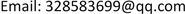VSP作为一种地球物理勘探方法在油田勘探开发中扮演着越来越重要的角色。它通过井中垂直排列上的波场响应，求地球物理参数、速度场信息、层位识别、井旁构造形态和地层岩性特征等，解决这些反问题的能力得到很大提高。同时为了更好的解决反问题首先也要处理好正问题—VSP正演模拟。下文以XX井为例，综合应用地震资料，测井资料，分别进行了基于射线理论和基于波动方程的两种VSP正演模拟的实现过程，并结合野外实际采集资料对比了二者方法的差异和优劣势。 As a geophysical exploration method, VSP plays an increasingly important role in oil field exploration and development. Through the response of wave field on the vertical arrangement of wells, it can obtain geophysical parameters, velocity field information, horizon identification, well-side structural and lithologic characteristics of strata, etc. The ability to solve these inverse problems has been greatly improved. In order to solve the inverse problem, we must also deal with the positive problem-VSP forward modeling. In this paper, taking well XX as an example, two VSP forward modeling methods based on ray theory and wave equation are implemented by using seismic data and logging data. The differences and advantages and disadvantages of the two methods are compared with the field data.

VSP正演模拟，射线理论，波动方程，射线追踪，正演道集记录, VSP Forward Modeling Ray Theory Wave Equation Ray Tracing Forward Track Record

1中国石油东方地球物理公司新兴物探开发处，河北 保定

2中国石油东方地球物理公司西南物探研究院，四川 成都

3中国石油西南油气田分公司勘探事业部，四川 成都VSP作为一种地球物理勘探方法在油田勘探开发中扮演着越来越重要的角色。它通过井中垂直排列上的波场响应，求地球物理参数、速度场信息、层位识别、井旁构造形态和地层岩性特征等，解决这些反问题的能力得到很大提高。同时为了更好的解决反问题首先也要处理好正问题—VSP正演模拟。下文以XX井为例，综合应用地震资料，测井资料，分别进行了基于射线理论和基于波动方程的两种VSP正演模拟的实现过程，并结合野外实际采集资料对比了二者方法的差异和优劣势。1. 前言

2. 射线理论2.1. 基本方程

ρ ∂ 2w ∂ 2 t = ( λ + μ ) ∇ ( ∇ ⋅ w ) + μ ∇ 2 w + ∇ λ ( ∇ ⋅ w ) + ∇ μ × ( ∇ × w ) + 2 ( ∇ μ ⋅ ∇ ) w (1)

∇ = i → ∂ ∂ x + j → ∂ ∂ y + k → ∂ ∂ z (2)

∇ μ = i → ∂ u ∂ x + j → ∂ u ∂ y + k → ∂ u ∂ z (3)

∇ ⋅ W = i → ∂ Wx ∂ x + j → ∂ Wy ∂ y + k → ∂ Wz ∂ z (4)

∇ × W = | i → j → k → ∂ x ∂ y ∂ z W x W y W z | (5)

W = exp [ j ω ( t − τ ) ] ∑ k = 0 ∞ ( j ω ) − k W k (6)

{ N ( W 0 ) = 0 N ( W 1 ) − M ( W 0 ) = 0 N ( W k ) − M ( W k − 1 ) + L ( W k − 2 ) = 0 ( k ≥ 2 ) (7)

(7)式中

{ N ( W k ) = − ρ W k + ( λ + μ ) ( W k ⋅ ∇ τ ) ∇ τ + μ ( Δ τ ) 2 W k M ( W k ) = ( λ + μ ) [ ∇ ( W k ⋅ ∇ τ ) + Δ τ ( Δ ⋅ W k ) ] + μ [ 2 ( ∇ τ ⋅ ∇ ) W k + ( ∇ 2 τ ) W k ]                             + ∇ λ ( W k ⋅ Δ τ ) + ∇ μ × ( ∇ τ × W k ) + 2 ( Δ μ ⋅ Δ τ ) W k L ( W k ) = ( λ + μ ) ∇ ( ∇ ⋅ W k ) + μ ∇ 2 W k + ∇ λ ( ∇ ⋅ W k ) + ∇ μ × ( ∇ × W k ) + z ( ∇ μ ⋅ ∇ ) W k (8)

V p = [ ( λ + 2 μ ) / ρ ] 1 / 2 (9)

V s = [ μ / ρ ] 1 / 2 (10)

2.2. 试射迭代方法

{ h ( i 0 , j 0 ) = H g ( i 0 , j 0 ) = G (11)

h和g为射线到达点的坐标，它是 i 0 和 j 0 的函数；

H和G是期望的射线到达点的坐标。

∂ h ∂ i 0 , ∂ h ∂ j 0 , ∂ g ∂ i 0 , ∂ g ∂ j 0 (12)

[ ∂ h ∂ i 0 ∂ h ∂ j 0 ∂ g ∂ i 0 ∂ g ∂ j 0 ] [ i 0 ( n + 1 ) − i 0 ( n ) j 0 ( n + 1 ) − j 0 ( n ) ] = [ H − h ( i 0 ( n ) , j 0 ( n ) ) G − g ( i 0 ( n ) , j 0 ( n ) ) ] (13)

3. 波动方程理论

3.1. 方法原理

U x x + U z z = V − 2 ( x , z ) U x z (14)

U k , j i = 2 ( 1 − 2 A 2 ) U k , j i − 1 − U k , j i − 1 + A 2 [ U k + 1 , j i − 1 + U k − 1 , j i − 1 + U k , j + 1 i − 1 + U k , j − 1 i − 1 ] (15)

A = V ( x k , z j ) Δ t / h (16)

U ( x k , z j , t i ) = 2 ( 1 − 2 A 2 ) U ( x k , z j , t i − 1 ) − U ( x k , z j , t i − 2 )     + A 2 [ U ( x k + 1 , z j , t i − 1 ) + U ( x k − 1 , z j , t i − 1 ) + U ( x k , z j + 1 , t i − 1 ) + U ( x k , z j − 1 , t i − 1 ) ] (17)

4. 应用实例

4.1. 资料准备

4.2. 地质模型建立

4.3. 观测系统布设

Well XX observation syste

SP13260-1010326197.84
SP23260-10103261521.77

4.4. 射线追踪

4.5. 正演道集记录

5. 结论